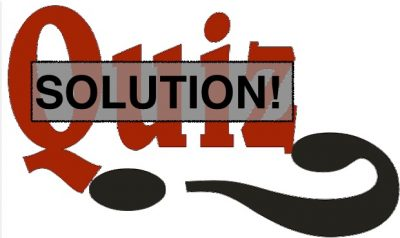# July 2020 Quiz SOLUTIONSince July is now over, here is the solution to the July 2020 WIARC Quiz. The monthly quiz will take a break for August.

### July Quiz Solution

Solution:

This quiz dealt with antenna feed-lines, impedances and SWR. To refresh your memory, he is the scenario:

An amateur radio operator has build a loop antenna for the 20m HF band. Using simulations and confirmation with an antenna analyzer, it has been determined that, at 14.1 MHz, the feed-point impedance (directly at the antenna) is 110 ohm with negligible reactive component (i.e. it is resonant at that frequency). The location of the antenna requires a feed-line length of at least 30 ft (9.1m).

Which of the following feed-line types/length combinations would be most appropriate to feed this antenna without the use of an antenna tuner or trans-match?

• A) 30 ft (9.1 m) of LMR400 (Zo = 50 ohms, 84% velocity factor) because it’s a good low-loss coax.
• B) 33 ft (10m) of any type of feed line because that length is 1/2 wavelength on 20m and that’s always the best length for any feed-line.
• C) Any length of twin-lead “window” line (Zo = 450 ohms, 91% velocity factor) because it propagates faster than coax and has lower loss.
• D) 35 ft (10.7m) of RG11 coax (Zo = 75 ohm, 67% velocity factor) because this length represents 3/4 of a wavelength which will transform the antennas’s 110 ohm impedance to 51 ohms (almost perfect) at the transceiver according to the formula Z1 x Z2=Zo^2
• E) 30 ft of CAT-5, or CAT-6 UTP ethernet cable because its characteristic impedance is 100 ohm, which will produce lower SWR than any of the other choices, and low SWR is all that matters for antenna system performance

The most correct answer is D). A transmission line with an electrical length equal to an odd number of quarter-wavelengths acts as an impedance transformer when there is standing wave, where the input impedance to the line Z1 and load impedance Z2 are such that Z1 x Z2 = Zo^2. So,  Z1 = Zo^2 / Z2 = 5625 / 110 = 51 ohms.